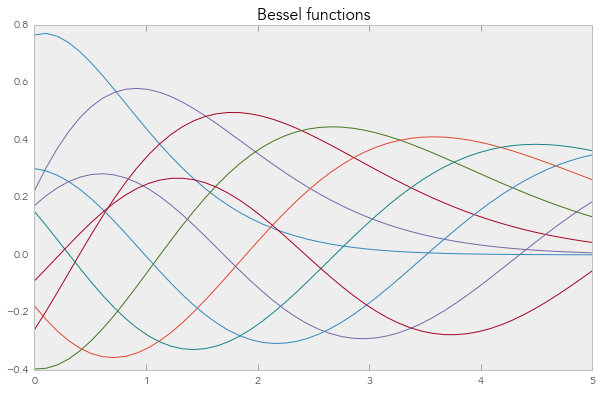# Simple animations Using clear_output¶

Sometimes you want to clear the output area in the middle of a calculation. This can be useful for doing simple animations. In terminals, there is the carriage-return ('\r') for overwriting a single line, but the notebook frontend can clear the whole output area, not just a single line.

To clear output in the Notebook you can use the clear_output function.

## Simple example¶

Here we show our progress iterating through a list:

In :
import sys
import time

In :
from IPython.display import display, clear_output
for i in range(10):
time.sleep(0.25)
clear_output()
print(i)
sys.stdout.flush()

9


## AsyncResult.wait_interactive¶

The AsyncResult object has a special wait_interactive() method, which prints its progress interactively, so you can watch as your parallel computation completes.

This example assumes you have an IPython cluster running, which you can start from the cluster panel

In :
from IPython import parallel
rc = parallel.Client()

amr = view.map_async(time.sleep, [0.5]*100)

amr.wait_interactive()

 100/100 tasks finished after    6 s
done


## Matplotlib example¶

You can also use clear_output() to clear figures and plots.

In :
%matplotlib inline
import numpy as np
import matplotlib.pyplot as plt

In :
from scipy.special import jn
x = np.linspace(0,5)
f, ax = plt.subplots()
ax.set_title("Bessel functions")

for n in range(1,10):
time.sleep(1)
ax.plot(x, jn(x,n))
clear_output()
display(f)

# close the figure at the end, so we don't get a duplicate
# of the last plot
plt.close()